• 正交变换
正交变换一般欧氏空间中的正交变换1 定义2 欧氏空间中的正交变换的刻伐n维欧氏空间中的正交变换
一般欧氏空间中的正交变换
1 定义2 欧氏空间中的正交变换的刻伐n维欧氏空间中的正交变换展开全文• 文章目录双正交关系、对偶基、正交基、正交变换正交变换的性质 《数字信号处理——理论、算法与实现》第二版 双正交关系、对偶基、正交基、正交变换 正交变换的性质
文章目录双正交关系、对偶基、正交基、正交变换正交变换的性质其他链接

《数字信号处理——理论、算法与实现》第二版

双正交关系、对偶基、正交基、正交变换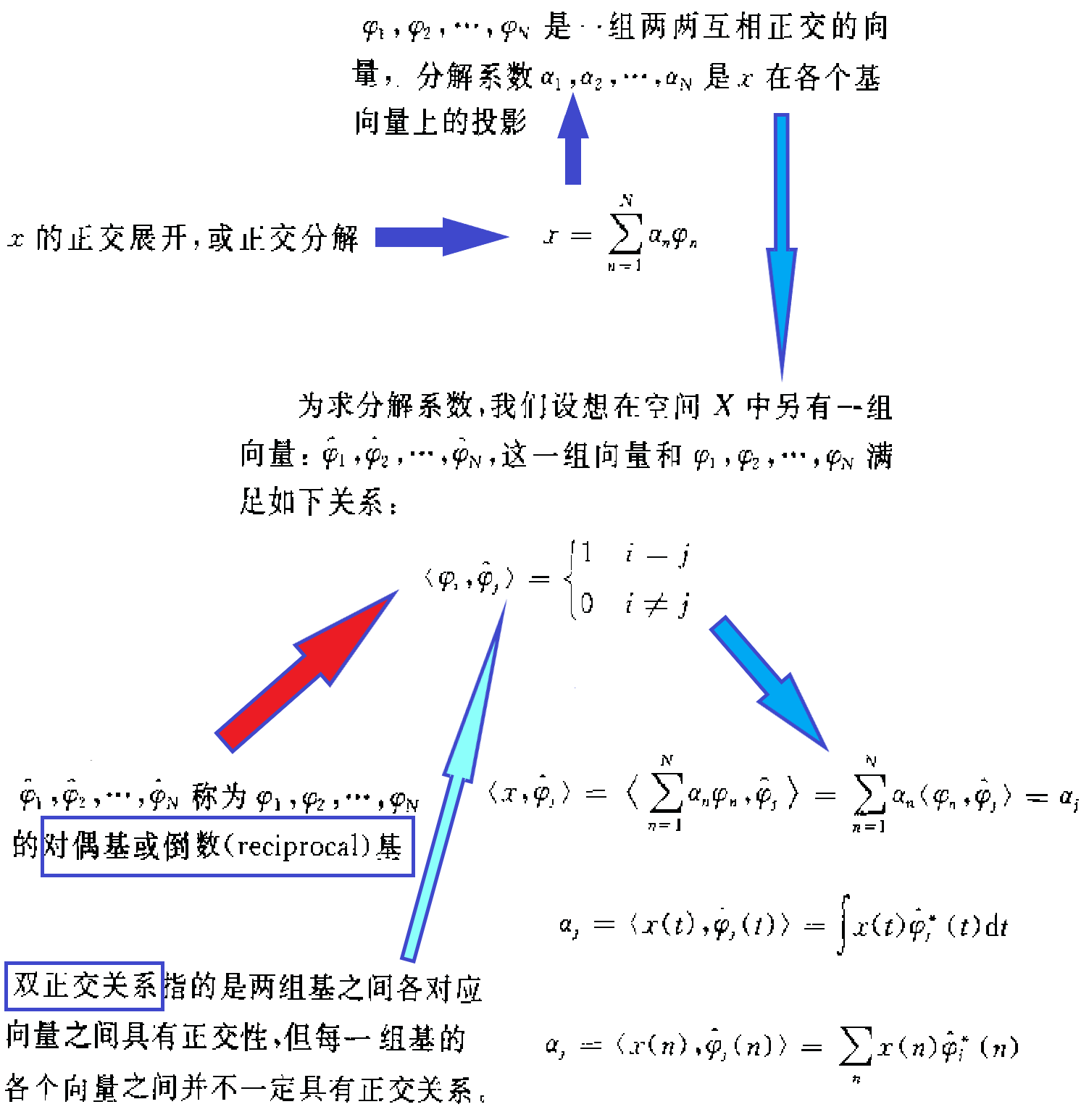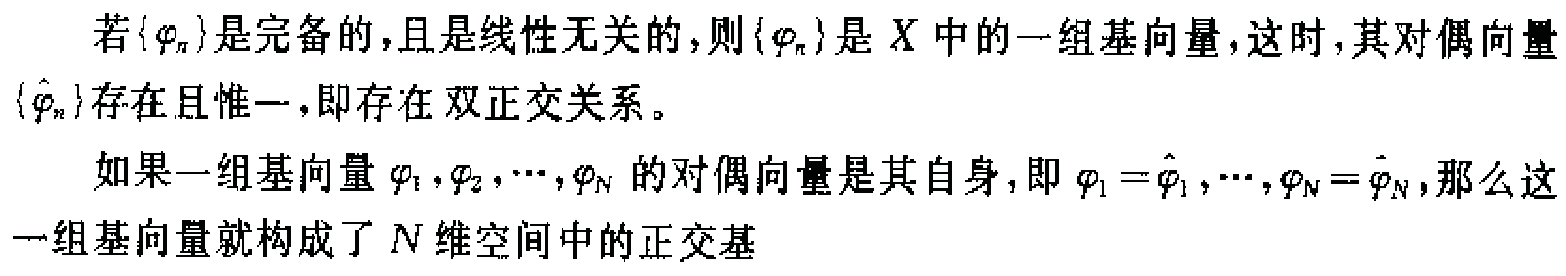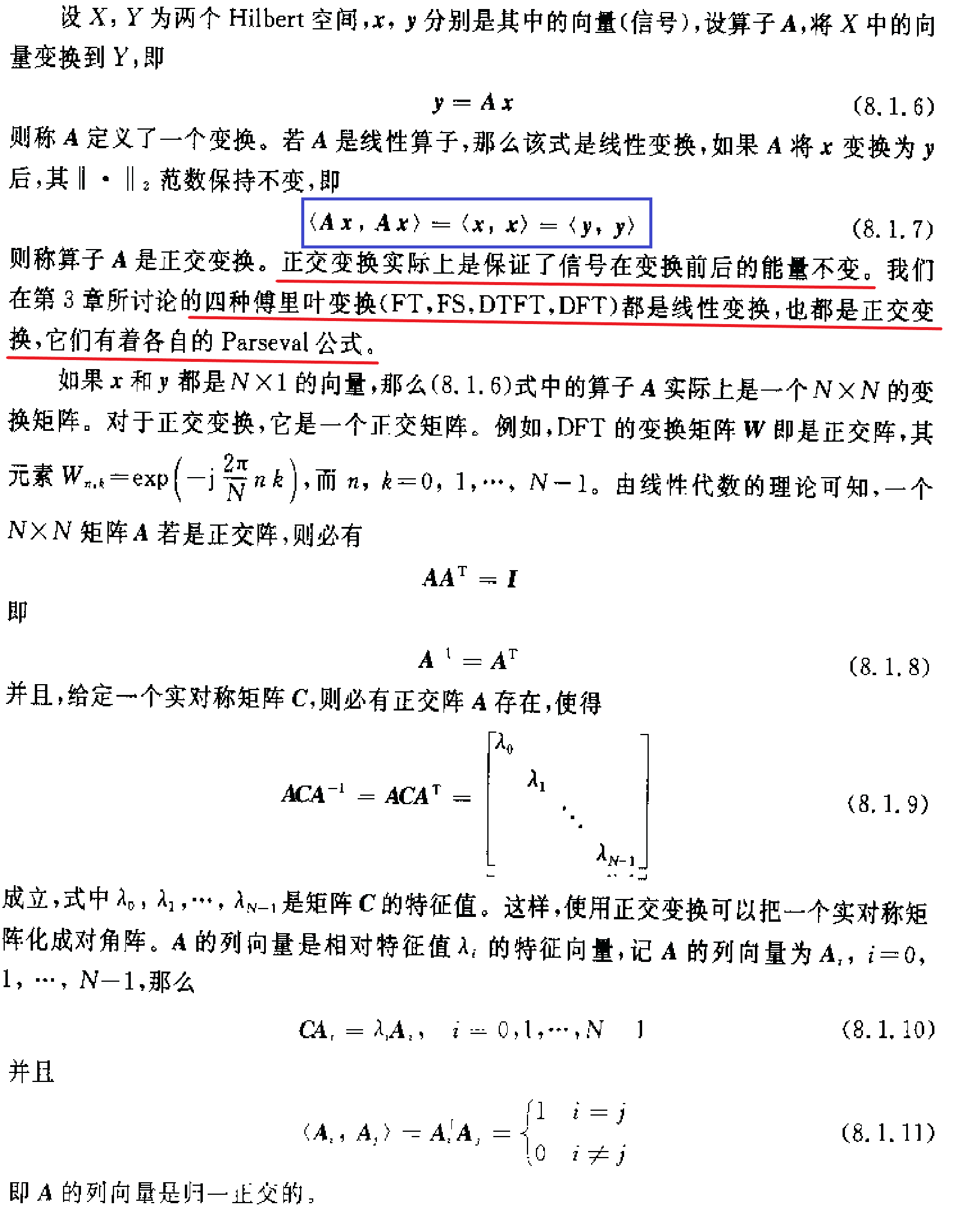正交变换的性质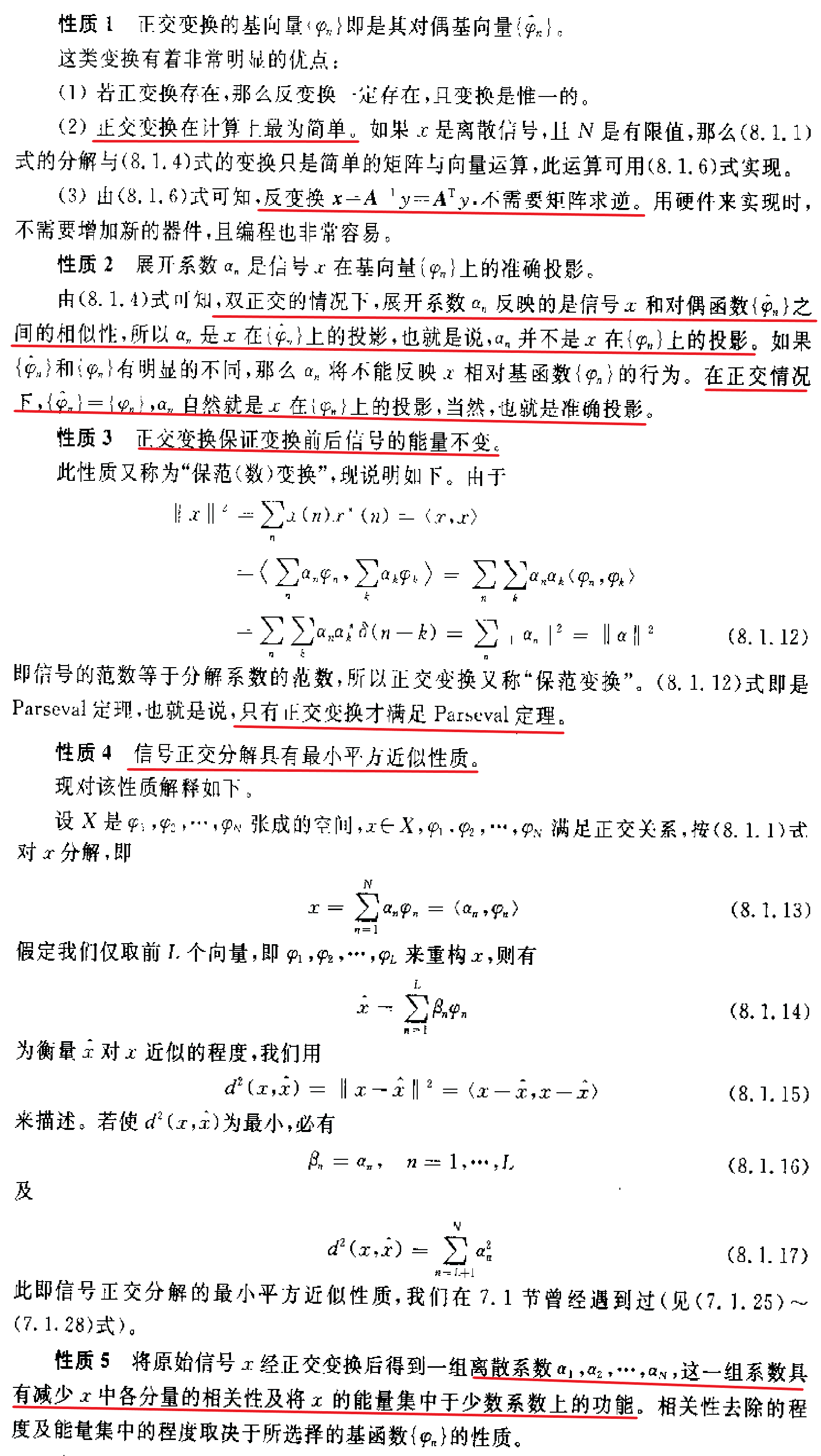其他链接

什么叫正交变换?为什么要正交变换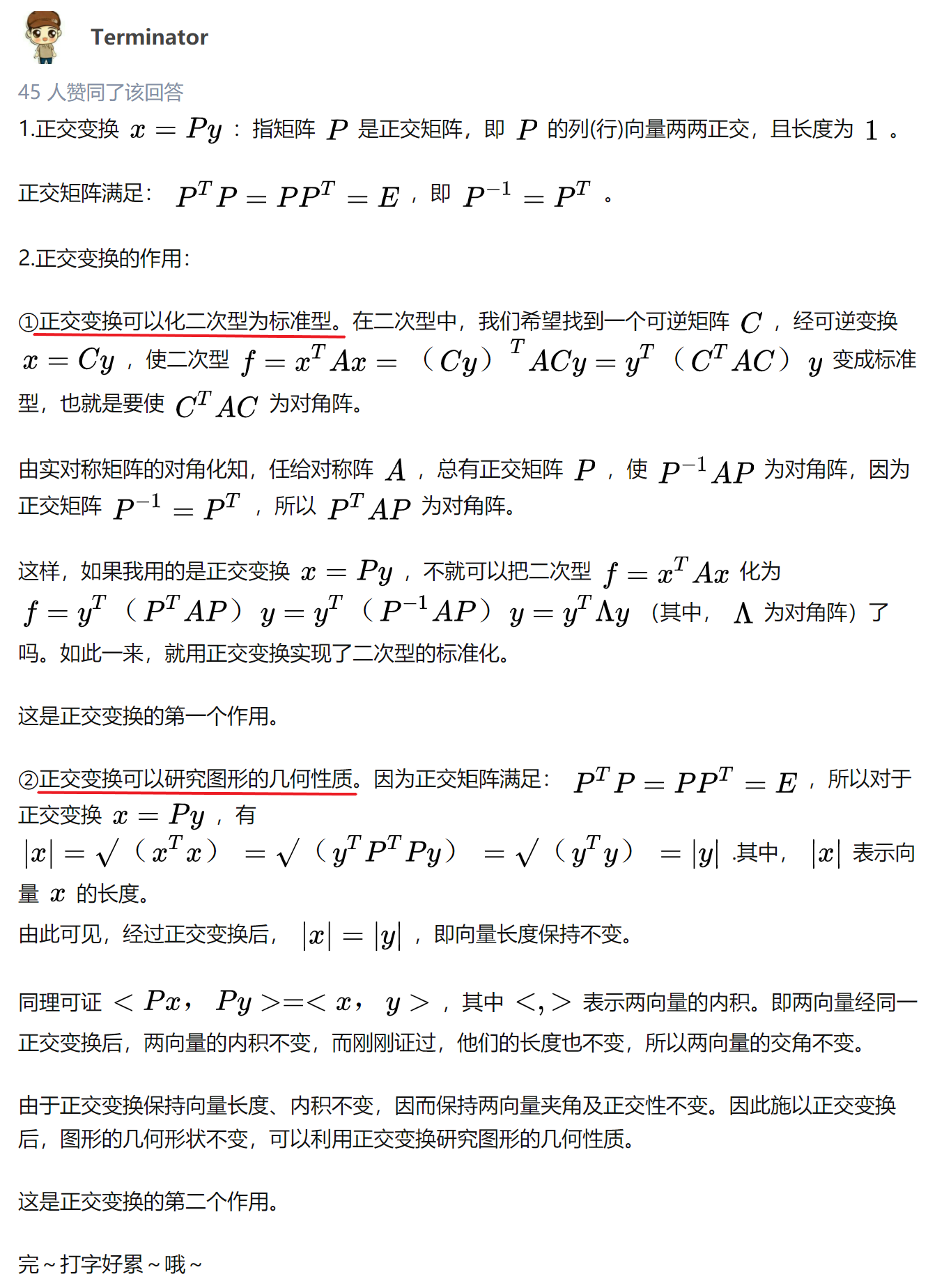展开全文• 正交变换VS2017编译通过，可直接运行 包括 傅立叶变换 离散余弦变换 沃尔什变换
• VC图像正交变换，VS2015编译通过，离散傅里叶变换，离散余弦变换，沃尔什哈达玛，离散K-L变换，SVD变换，小波变换
• 常用的图像正交变换的代码，傅里叶变换，离散余弦变换
• 数字图像处理正交变换实验傅里叶变换dct变换频谱图数字图像处理
• 正交变换 VS2017编译通过，可直接运行 包括一维傅立叶变换 二维离散余弦变换 沃尔什变换
•计算机视觉
• 正交变换（傅里叶变换、Z变换） ​ 信号分解方法多种多样，我们可将信号分解为直流分量+交流分量、偶分量+奇分量、实部分量+虚部分量、脉冲分量、正交分量等多种形式。其中一个较复杂而又有重要意义的分解方法便是...
正交变换（傅里叶变换、Z变换）

​	信号分解方法多种多样，我们可将信号分解为直流分量+交流分量、偶分量+奇分量、实部分量+虚部分量、脉冲分量、正交分量等多种形式。其中一个较复杂而又有重要意义的分解方法便是将信号分解为正交分量，我们把这个过程称作：信号的正交分解（正交变换）。
​	将信号正交分解之后，可以用于：

方便处理
便于抽取特性
数据压缩

​
​	首先有一个问题——什么是正交？
​	在线性代数中我们了解过，向量的正交指的是$\vec{a}\cdot\vec{b} = 0$——两个向量的内积为0，意味着两个向量在对方上的投影为0，即可认为这两个向量互不相关互不影响。显然，向量的正交对应的是正交的离散形式$\sum_{i=0}^n a_i * b_i = 0$。
​	然而，从信号的角度，许多信号其实是连续的，由连续的函数所表示，那么我们又该如何定义正交的连续形式呢？由此，我们引入连续函数的概念：如果在区间 ($t_1, t_2$) 上，函数 $f_1$(t) 和 $f_2$(t) 互不含有对方的分量，则称 $f_1$(t) 与 $f_2$(t) 在($t_1, t_2$)上正交。 即：
$ = \int_{t_1}^{t_2}f_1(t)f_2(t)dt = 0$
​	并有定理：

任一函数 f (t)在 ($t_1, t_2$) 上可表示为正交函数集内函数 的线性组合。
$f(t) \approx \sum_{n=1}^Nc_ng_n(t)$

​
​	同时，用于正交变换的方法也多种多样：

傅里叶变换 Fourier Transform

离散余弦变换 Discrete Cosine Transform

沃尔希-哈德玛变换 Walsh-Hadamard Transform

斜变换 Slant Transform

哈尔变换 Haar Transform

离散小波变换 Discrete Wavelet Transform

离散K-L变换 Discrete Karhunen-Leave Transform

奇异值分解SVD变换 Singular-Value Decomposition

Z变换

​	本次学习心得中，我将主要介绍自主学习了解的傅里叶变换及其衍生Z变换这两种正交变换方法。

傅里叶变换
对傅里叶变换的理解
​	法国数学家让·巴普蒂斯·约瑟夫·傅里叶男爵发现，任何周期函数都可以用正弦函数和余弦函数构成的无穷级数来表示：
$f(t) = \frac{a_0}{2} + \sum_{n=1}^{\infty}[a_n\cos(nw_0t) + b_n\sin(nw_0t)]$​	可以将*f(x)*理解为由如下正交基表示成的向量：
$\{1,\cos(\frac{2\pi n}{T}x),\sin(\frac{2\pi n}{T}x)\}$
​	那么上面的式子就可以解读为：​	令$w_n = \frac{2\pi n}{T}$ ,从三角函数的意义来说，$w_n$就对应着该三角函数的角频率，对于信号，则可看作是一个正弦波对应的角频率。
​	我们将x（通常代表时间t）作为自变量可以画出信号f(x)在时域上的函数图像：​	而将频率$w_n$作为自变量我们同样也可以画出信号f(x)在频域上的函数图像：​	将二者综合我们可以看到这样一个图像：​	那么，我们从信号的角度可以这么认为——傅里叶级数展开的核心意义在于：将一个周期信号（时域上看只有一个）分解为了多个不同频率下的相互正交（相互独立、互不干扰）的信号。傅里叶级数展开的本质是信号由时域到频域
​	我们注意到，傅里叶级数展开有一个前提条件：函数（信号）必须是周期的！但在生活研究中，许多信号显然都无法符合具有周期性这个要求。那么，对于非周期函数（信号），我们该如何实现时域到频域的分解变化呢？
​	例如：下图这个函数，由于并非一个周期函数，无法写出它的傅里叶级数。​	然而，我们可以拓宽一下思路，没有多个连续周期，但我们可以将函数的整个定义域（$-\infty , \infty$)看作是唯一的一个周期T：
$T \longrightarrow \infty$
​	将T慢慢变大我们可以观察到：​	频域上看，这些频率就会变得稠密，直至连续，变为一条频域曲线：​	傅立叶变换就是，让$T=\infty$，求出上面这根频域曲线的过程。即傅里叶变换是傅里叶级数展开对应非周期函数（信号）的拓展延伸。
​	下面是傅里叶变换的数学公式推导：
​	前面所述的傅里叶级数：
$f(t) = \frac{a_0}{2} + \sum_{n=1}^{\infty}[a_n\cos(nw_0t) + b_n\sin(nw_0t)],a_0\in\mathbb{R}$
​	通过欧拉公式可以将其等价变换为复数形式：
$f(x) = \sum_{n = -\infty}^{\infty}c_n\cdot e^{i\frac{2\pi nx}{T}}$
​	复数形式下，将其看作向量的话正交基则为：
$\{e^{i\frac{2\pi nx}{T}}\}$
​	将周期T推向无穷时：
$\begin{cases} f(x) = \sum_{n=-\infty}^{\infty}c_n\cdot e^{-i\frac{2\pi nx}{T}} \\ T=\infty \end{cases}\Longrightarrow f(x) = \int_{-\infty}^{\infty}F(\omega)e^{i\omega x}dx$
​	由此可推出$F(\omega)$：
$\begin{cases} c_n = \frac{1}{T}\int_{x_0}^{x_0+T}f(x)\cdot e^{-i\frac{2\pi nx}{T}}dx \\ T = \infty \end{cases} \Longrightarrow F(\omega) = \frac{1}{2\pi}\int_{-\infty}^{\infty}f(x)e^{-i\omega x}dx$
​	$F(\omega)$就是傅里叶变换，得到的就是频域曲线。
​	$f(x)$与$F(\omega)$二者成为傅里叶变换对，可以相互转换：
$F(\omega)=\int_{-\infty}^{\infty}f(t)e^{-j\omega t}dt \iff f(t) = \frac{1}{2\pi}\int_{-\infty}^{\infty}F(\omega)e^{j\omega t}d\omega$
​	可以这么说，二者是同一个数学对象的两种形式，一个是函数，一个是向量。
傅里叶变换的性质及其应用举例
​	连续傅里叶变换的一些性质

模、幅度函数、相角
$F(u) = R(u)+jI(u)=|F(u)|e^{j\phi(u)}$
模：$F(u)=\sqrt{R^2(u)+I^2(u)}$ （也称为幅度函数，傅里叶谱，频谱）

相角：$\phi(u)=tan^{-1}[\frac{I(u)}{R(u)}]$
函数$f(x)$:幅度函数：双变量函数$f(x,y)$的傅里叶变换
$\Im\{f(x,y)\} = F(u,v)\\ \Im^{-1}\{F(u,v)\} = f(x,y)$

对称性

傅里叶变换后得到实奇部与虚偶部：$F(u) = F_e(u)-jF_o(u)$
故$F(u)$具有共轭对称性：$F(u) = F^*(-u)$

加法原理
$\Im\{f(x)+g(x)\} = F(u)+G(u)$
且由加法定义可推出：
$\Im\{cf(x)\} = cF(u)$
图像展示：平移原理
$\Im\{f(x-a)\}=e^{j2\pi ua}F(u)$

相似性原理(展缩性质)
$\Im\{f(ax\}=\frac{1}{|a|}F(\frac{u}{a})$
图像展示：Rayleigh’s原理

$energy = \int_{-\infty}^{\infty}|f(x)|^{2}dx = \int_{-\infty}^{\infty}|F(u)|^2du$

​	离散傅里叶变换的一些性质

可分离性
$F(u,v) = \frac{1}{N}\sum_{x=0}^{N-1}e^{-j2\pi ux/N}\cdot\sum_{y=0}^{N-1}f(x,y)e^{-j2\pi vy/N}\\f(x,y) = \frac{1}{N}\sum_{u=0}^{N-1}e^{-j2\pi ux/N}\cdot\sum_{v=0}^{N-1}F(u,v)e^{-j2\pi vy/N}$

线性性质
$\Im\{af(x,y)+bg(x,y)\} = a\Im\{f(x,y)\}+b\Im\{g(x,y)\}$

比例性
$\Im\{f(ax,by)\} = \frac{1}{|ab|}F(\frac{u}{a},\frac{v}{b})$

周期性
$F(u) = F(u+N)$

​
​	快速傅里叶变换
​	利用共轭性质将逆离散傅里叶变换变为其共轭数的正离散傅里叶变换。
​	傅里叶变换应用举例
​	图(a)为一幅受正弦干扰模式覆盖的图像。 图(b)是(a)的傅立叶频谱幅度图， 其上有一对较明显的脉冲白点（正交分解后便于处理）。利用带阻滤波器除掉亮点。然后取傅立叶反变换，就可得到图(d)和 (f)所示的恢复效果。Z变换
Z变换的引入原因及其定义
​	首先，我们先来看看Z变换的定义：
​	序列$x(n)$的$z$变换$X(z)$定义为：
$X(z) = \sum_{n = -\infty}^{\infty}x(n)z^{-n}$
​	表达式中的$z$是可由我们自由选取的，因此，通过改变$z$的值，我们总能够找到一个$z$使得$\sum_{n = -\infty}^{\infty}|x(n)|z^{-n}<\infty$成立，即使$X(z)$这个级数收敛。对给定的序列，使z变换收敛的那些$z$值就称为z变换的收敛域，缩写ROC。
​	同理我们可以知道，无穷项之和不可能总是有限的，因此傅里叶变换的幂级数不是对所以序列都收敛。这也就是我们引入Z变换的原因——傅里叶变换不是对所有序列都收敛，因此我们需要一个能包括更广泛信号的傅里叶变换的推广形式——Z变换。
​	下面来介绍一些常见的基本Z变换：

离散冲激信号：$\delta(n)$
$X(z)=\sum_{n=0}^{\infty}\delta(n)z^{-n}=1$

阶跃信号：$u(n)$($|z|>1$才能收敛)
$X(z) = \sum_{n=0}^{\infty}u(n)z^{-n} = \frac{z}{z-1}$

斜线信号：$x(n) = n u(n)$
$X(z) = \sum_{n=0}^{\infty}nz^{-n}=\frac{z}{(z-1)^2}$

指数序列：$x(n) = a^nu(n)$($|z|>|a|$才能收敛)
$X(z) = \sum_{n=0}^{\infty}a^nz^{-n} = \frac{z}{z-a}$

Z变换的性质及其应用
​	Z变换的一些性质

线性
若$x(n)$的Z变换为$X(z)$，$y(n)$的Z变换为$Y(z)$
则$a\cdot x(n) + b\cdot y(n)$的Z变换为$a\cdot X(z) + b\cdot Y(z)$

时移
若$x(n)$的Z变换为$X(z)$
则$x(n-m)$的Z变换为$z^{-m}*X(z)$

初值定理
若$x(n)$的Z变换为$X(z)$
则$x(0) = \lim_{z\to \infty}X(z)$

Z域微分
若$x(n)$的Z变换为$X(z)$
则$nx(n)$的Z变换为$-z\frac{d[X(z)]}{dz}$

终值定理
若$x(n)$的Z变换为$X(z)$
则：
$\lim_{n\to \infty}x(n) = \lim_{z\to1}[(z-1)X(z)]$

​	Z变换应用举例——消除匀速直线运动
​​	最终效果：展开全文信号处理
• 讨论准正交变换正交变换的关系,以及准正交变换的性质。获得：若aO,...,am与β1,…,βm是n维欧氏空间V中向量组,则存在一个准正交变换成δ,使δ(ai)＝βi当且仅当存在c∈R+,使c
•研究论文
• 图像信号的正交变换正交变换的概念，正交变换的优点及应用。
• 指的是把图像中的各个像素通过一种数学变换（如傅立叶变换、正交变换等）从一种空间变换到一种空间（如频率域、正交矢量空间），信号在时域或空域信息冗余度大，而变换后参数之间相关性很小或者不相关，减少了数据量...
变换？指的是把图像中的各个像素通过一种数学变换（如傅立叶变换、正交变换等）从一种空间变换到一种空间（如频率域、正交矢量空间），信号在时域或空域信息冗余度大，而变换后参数之间相关性很小或者不相关，减少了数据量。

利用人的视觉特性—对高频细节不敏感，滤除高频系数、保留低频系数，对变换后的信号进行量化与编码操作，达到数据压缩的效果。最后再在接收端对信号译码、反变换恢复原图。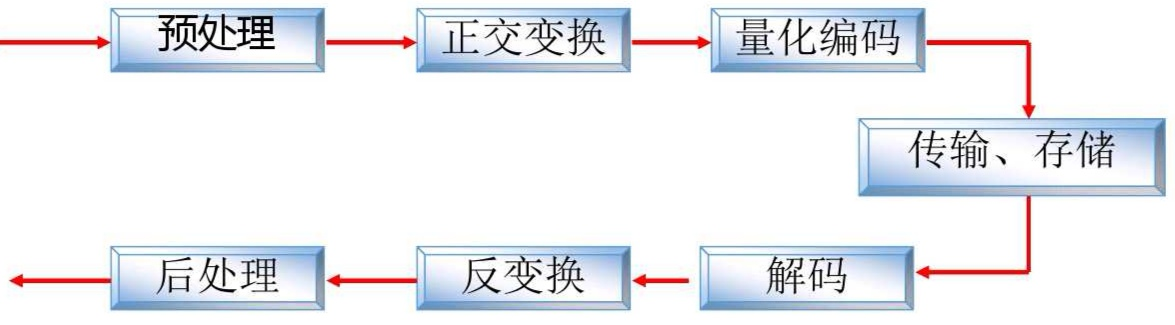预测编码可以去除图像数据的时间和空间上的冗余，而变换编码则是希望数据在新的空间中尽可能相互独立，能力更加集中。但我们为什么要选择正交变换呢？让我们看看正交变换的优势所在。

极限熵的保持
正交变换本身不会丢失信息，可以用传递变换系数实现信息传递。

能量保持
变换域中信号能量与原来空间域中的信号能量相等。

去（解）相关
正交变换可以将强相关的空间样值变为不相关或弱相关的变换系数，去除存在于相关性之中的数据冗余度。

能量重新分配与集中
变换域系数与DPCM法相同，零与小幅值系数占绝大多数，但DPCM的幅度分布在全空间均可能，要对每一个残差编码，而变换法则按统计规律集中分布在一定区域上，舍弃能量较小者或给其分配较少的位数，压缩数据率。

变换域系数的编码有区域编码、阈值编码、系数量化三种方式。其中区域编码只对规定区域内的变换系数进行量化编码，而编码区里域的形状取决于压缩比、变换方式、变换块大小等因素。变换域信号能量集中在矩阵左上部，且左上角元素集中能量最大，因此我们只对变换域左上部区域变换系数进行编码传输，对右下角不进行编码传输。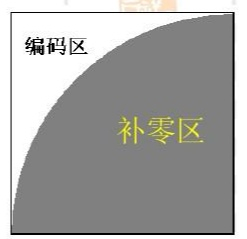这样的编码方式虽然简单并有利于限制误码扩散，但是它会严重损失图像质量，造成边缘模糊等问题。因此可以采用阈值编码，多数低频成分仍被编码输出，而少数超过阈值的高频成分也被编码输出，来弥补区域编码的不足。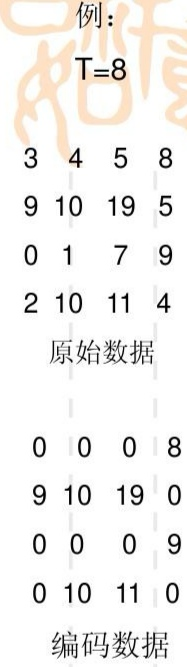展开全文• 为了解决容积卡尔曼滤波(CKF)算法在处理高维问题时出现的非局部采样问题,提出基于采样点正交变换的改进CKF算法(TCKF).从数值积分近似角度导出无迹卡尔曼滤波(UKF)和CKF两种近似滤波算法,并指出CKF只是UKF的一个特例;...
• 如果对于任意向量u{u}u和v{v}v，其内积等于转换后向量T(u)T({u})T(u)和T(v)T({v})T(v)的内积，则该转换称之为正交变换 即：⟨u,v⟩=⟨T(u),T(v)⟩\langle{u}, {v}\rangle=\langle T({u}), T({v})\rangle⟨u,v⟩=⟨T...
• 针对传统数字正交变换存在A/D采样速率要求比较高导致后续的数字低通滤波成为瓶颈、运算数据量大、需要正交本振等方面的不足，提出了一种基于软件无线电多相滤波的数字正交变换技术。首先分析基于多相滤波的数字正交...
• 数字图像处理 正交变换 标准正交基 数字图像处理 正交变换 标准正交基
• 文章目录正交变换正交补正交投影参考 正交变换 约定 :V: V:V 是 nnn 维欧氏空间. 正交变换: 线性变换 A\mathcal{A}A 满足 (Aα,Aβ)=(α,β),∀α,β∈V.(\mathcal{A} \alpha, \mathcal{A} \beta)=(\alpha, \beta), ...
•矩阵
• C++实现灰度图像正交变换，包括傅里叶变换、离散余弦变换、沃尔什变换，小波变换（bmp灰度图像），VC6.0里运行无误
• 什么是正交变换正交变换为何要满足下列条件？正交变换研究什么？ 1 表象 2 正交变换：研究”长度不变“ 3 性质 角度，长度，面积不变 4 基本形式 （1）平移变换 （2）旋转变换 （3）轴反射变换 ...高等几何 三维重建
• 图像的正交变换源码 傅里叶变换 离散余弦变换 沃尔什变换...